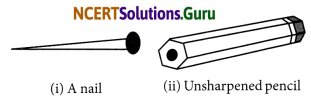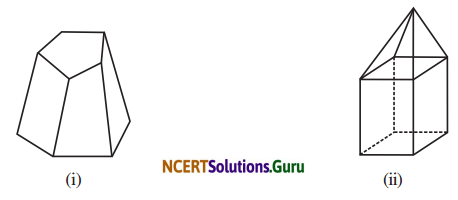# NCERT Solutions for Class 8 Maths Chapter 10 Visualizing Solid Shapes Ex 10.3

These NCERT Solutions for Class 8 Maths Chapter 10 Visualizing Solid Shapes Ex 10.3 Questions and Answers are prepared by our highly skilled subject experts.

## NCERT Solutions for Class 8 Maths Chapter 10 Visualizing Solid Shapes Exercise 10.3

Question 1.
Can a polyhedron have for its faces.
(i) 3 triangles
(ii) 4 triangles
(iii) a square and four triangles?
(i) No, it is not possible
(ii) Yes, four triangles can be the faces of a polyhedron.
(iii) Yes, a square and four triangles can be the faces.

Question 2.
Is it possible to have a polyhedron with any given number of faces?
(Hint: Think of a pyramid)
Possible only if the number of faces is greater than or equal to 4.Question 3.
Which are prisms among the following?(i) A nail
(ii) Unsharpened pencil
(i) No, a nail is not a prism.
(ii) Yes, unsharpened pencil is a prism.
(iii) No, table weight is not a prism.
(iv) Yes, box is a prism.

Question 4.
(i) How are prisms and cylinders alike?
(ii) How are pyramids and cones alike?
(i) A prism becomes a cylinder as the number of sides of its base becomes larger and larger.
(ii) A pyramid becomes a cone as the number of sides of its base becomes larger and larger.

Question 5.
Is a square prism same as a cube?
Explain:
No, it can be a cuboid also.Question 6.
Verify Euler’s formula for these solids.In the given figure,
F = 7, V = 10 and E = 15
F + V – E = 7 + 10 – 15 = 17 – 15
F + V – E = 2
Thus, Euler’s formula is verified.

(ii) In the given figure,
F = 9, V = 9 and E = 16
F + V- E = 9 + 9 – 16 = 18 – 16
Thus, Euler’s formula is verified.

Question 7.
Using Euler’s formula find the unknown.(i) Here V = 6 and E = 12
F + V – E = 2
F + 6 – 12 = 2
F – 6 = 2
F = 6 + 2 = 8

(ii) F = 5, E = 9
F + V – E = 2
5 + V – 9 = 2
V – 4 = 2
V = 2 + 4 = 6

(iii) Here F = 20 V = 12
F + V – E = 2
20 + 12 – E = 2
32 – E = 2
32 – 2 = E
30 = EQuestion 8.
Can a polyhedron have 10 faces, 20 edges and 15 vertices?